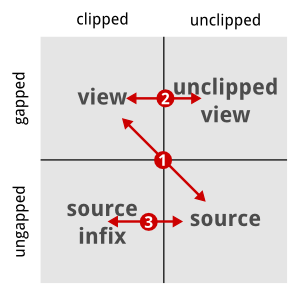# Computing Positions In Clipped Alignments¶

This page describes how to compute view and source positions in an unclipped and clipped Align.

## Position Computation Overview¶

There are four coordinate systems related to each gap object. One can consider the positions with and without gaps, both with and without clipping. The following picture and list show the easiest transformations between the coordinate systems.1. Translate between view (gapped clipped) position and source (ungaped unclipped) position using the functions toSourcePosition and toViewPosition.
2. Translate between clipped and unclipped gapped position by adding/subtracting clippedBeginPosition of the gaps object.
3. Translate between clipped ungapped and unclipped ungapped position by adding/subtracting beginPosition of the gaps object.

All other transformations are most easily done following one of the paths from the picture above.

## An Example¶

The following extensive example shows how to practically translate between the coordinate systems.

// Demo program for clipping with Gaps objects.

#include <iostream>

#include <seqan/sequence.h>
#include <seqan/align.h>

int main()
{
typedef seqan::Position<seqan::Gaps<seqan::CharString> >::Type TPos;

// Create sequence variable and gaps basd on sequence.
seqan::CharString seq("ABCDEFGHIJ");
seqan::Gaps<seqan::CharString> gaps(seq);

// Insert gaps, the positions are in (clipped) view space.
insertGaps(gaps, 2, 2);
insertGap(gaps, 6);
insertGap(gaps, 10);

// Print to stdout.
std::cout << "gaps\t" << gaps << "\n"
<< "seq \t" << seq << "\n\n";

// Print the begin and end positions in sequence space and the clipped
// begin and end positions in gap space.  We have no clipping, so no
// surprises here.
std::cout << "beginPosition(gaps)        == " << beginPosition(gaps) << "\n"
<< "endPosition(gaps)          == " << endPosition(gaps) << "\n"
<< "clippedBeginPosition(gaps) == " << clippedBeginPosition(gaps) << "\n"
<< "clippedEndPosition(gaps)   == " << clippedEndPosition(gaps) << "\n\n";

// Now, clip the alignment and again print the gaps, sequence and begin/end
// positions.  Note that the clipping positions are relative to the unclipped
// view.
setClippedBeginPosition(gaps, 3);
setClippedEndPosition(gaps, 10);

std::cout << "gaps\t" << gaps << "\n"
<< "seq \t" << infix(seq, beginPosition(gaps), endPosition(gaps)) << "\n\n";

std::cout << "beginPosition(gaps)        == " << beginPosition(gaps) << "\n"
<< "endPosition(gaps)          == " << endPosition(gaps) << "\n"
<< "clippedBeginPosition(gaps) == " << clippedBeginPosition(gaps) << "\n"
<< "clippedEndPosition(gaps)   == " << clippedEndPosition(gaps) << "\n\n";

// We can translate between the (clipped) gapped position (aka view) and
// the unclipped ungapped positions (aka) source using toSourcePosition()
// and toViewPosition().  Note that because of projection to the right of
// gaps, these operations are not symmetric.
std::cout << "4 view position => " << toSourcePosition(gaps, 4) << " source position\n"
<< "2 source position => " << toViewPosition(gaps, 2) << " view position\n\n";

// Translating between clipped gapped and unclipped gapped position can
// be done by adding/subtracting clippedBeginPosition(gaps).
std::cout << "3 clipped gapped => " << 3 + clippedBeginPosition(gaps) << " unclipped gapped\n"
<< "6 unclipped gapped => " << 5 - clippedBeginPosition(gaps) << " clipped gapped\n\n";

// Translating between clipped ungapped and unclipped ungapped position can
// be done by adding/subtracing beginPosition(gaps).  Since there are no
// gaps, this operation is symmetric.
std::cout << "3 clipped ungapped => " << 3 + beginPosition(gaps) << " unclipped ungapped\n"
<< "5 unclipped ungapped => " << 5 - beginPosition(gaps) << " clipped ungapped\n\n";

// Translating between gapped clipped position and ungapped clipped
// position and between gapped unclipped and ungapped unclipped positions
// has to be done using the translations above.
std::cout << "3 clipped gapped => " << toSourcePosition(gaps, 3) - beginPosition(gaps) << " clipped ungapped\n"
<< "4 unclipped ungapped => " << toViewPosition(gaps, 4) + clippedBeginPosition(gaps) << " unclipped gapped\n";

return 0;
}